# Plotting LMDZ output with Python¶

In this notebook, we demonstrate commands to visualize LMDZ output. We will use Python modules that may not be installed on your computer. We do not cover here the installation of these modules.

## Basic plotting using only the modules netCDF4 and matplotlib¶

In :
import netCDF4
import matplotlib.pyplot as plt


Let us take one of the history files from LMDZtrunk/modipsl/modeles/LMDZ/BENCH32x32x39.

One-dimensional plot. For example, temperature as a function of time, at 1st model level, 17th latitude, 17th longitude:

In :
f = netCDF4.Dataset("histhf.nc")
plt.plot(f["time_counter"][:] / 86400, f["temp"][:, 0, 16, 16])
plt.ylabel("temperature (K)")
plt.xlabel("time (days since Jan. 1st 1980)")

Out:
Text(0.5, 0, 'time (days since Jan. 1st 1980)')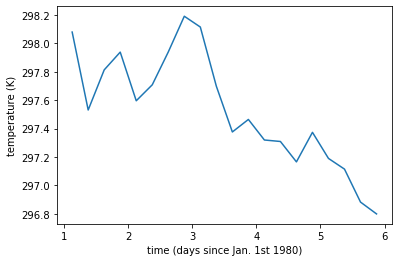## nc_time_axis¶

If you want a better representation of the time coordinate, you can use the module nc_time_axis. But first you need to correct the calendar, which does not conform to CF conventions. This is necessary to use the module nc_time_axis:

In :
if f["time_counter"].calendar == "360d":
f.close() # close before re-opening in writing mode

with netCDF4.Dataset("histhf.nc", "r+") as f:
f["time_counter"].calendar = "360_day"

f = netCDF4.Dataset("histhf.nc") # re-open in read-ony mode

In :
import nc_time_axis
time_counter = netCDF4.num2date(f["time_counter"][:], f["time_counter"].units,
f["time_counter"].calendar)
t = [nc_time_axis.CalendarDateTime(item, "360_day") for item in time_counter]
plt.plot(t, f["temp"][:, 0, 16, 16])
plt.ylabel("temperature (K)")
plt.gcf().autofmt_xdate()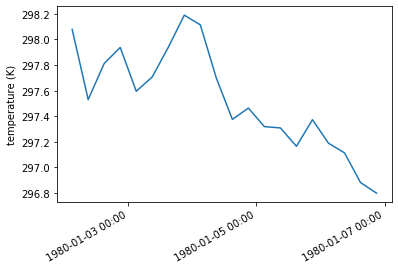## Contour plot with matplotlib only¶

Contour plot, for example, temperature as a function of latitude and longitude, at 1st time, 1st model level:

In :
plt.contourf(f["lon"][:], f["lat"][:], f["temp"][0, 0])
plt.colorbar(label = "temperature (K)")
plt.xlabel("longitude (degrees east)")
plt.ylabel("latitude (degrees north)")

Out:
Text(0, 0.5, 'latitude (degrees north)')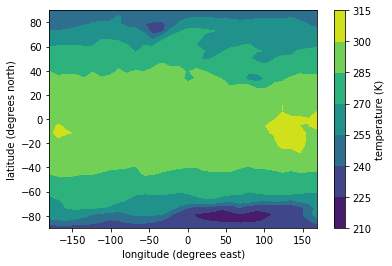## Basemap¶

If you want to add coastlines, you can use the module basemap:

In :
from mpl_toolkits import basemap
import numpy as np
m = basemap.Basemap(projection = "robin", lon_0 = 0)
lon_2d, lat_2d = np.meshgrid(f["lon"], f["lat"])
m.contourf(lon_2d, lat_2d, f["temp"][0, 0], latlon = True)
m.drawparallels(range(-80,81,20), labels=[1,0,0,0])
m.drawmeridians(range(-180,180,60), labels=[0,0,0,1])
m.colorbar(label = "temperature (K)")
m.drawcoastlines()

Out:
<matplotlib.collections.LineCollection at 0x154189f86880>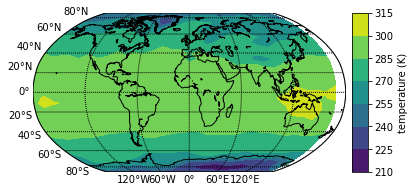## Cartopy¶

An alternative way of obtaining approximately the same result is with the module cartopy. cartopy is the official replacement for basemap, which is no longer maintained. So:

In :
import cartopy.crs as ccrs
ax = plt.axes(projection=ccrs.Robinson())
plt.contourf(lon_2d, lat_2d, f["temp"][0, 0],
transform = ccrs.PlateCarree())
ax.coastlines()
ax.set_global()
ax.gridlines(draw_labels = True)
plt.colorbar(label = "temperature (K)", orientation='horizontal')

Out:
<matplotlib.colorbar.Colorbar at 0x154180a29940>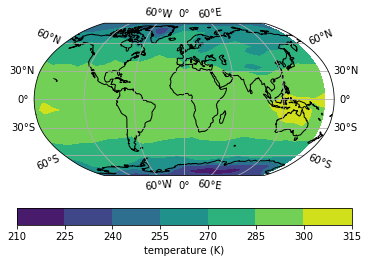## xarray¶

Instead of opening a NetCDF file with the module netCDF4, you can open it with xarray. xarray is actually built on top of netCDF4, it offers a higher-level interface to the data. For example, you can select a slice of the data by referring to the name of a dimension, instead of referring to the position of this dimension in the list of dimensions. Here is an example. But first, close the file opened with netCDF4:

In :
f.close()
import xarray as xr

In :
f = xr.open_dataset("histhf.nc")

In :
p = f.temp.isel(time_counter = 0, presnivs = 0).plot(subplot_kws={"projection": ccrs.Robinson()},
transform=ccrs.PlateCarree())
p.axes.coastlines()
p.axes.gridlines(draw_labels = True)

Out:
<cartopy.mpl.gridliner.Gridliner at 0x15417dff82e0>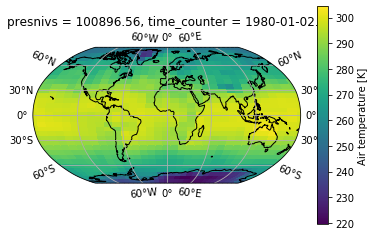In [ ]: